# Pipes and Cisterns - Concepts and Tricks Quant Notes | EduRev

## Quant : Pipes and Cisterns - Concepts and Tricks Quant Notes | EduRev

The document Pipes and Cisterns - Concepts and Tricks Quant Notes | EduRev is a part of the Quant Course Quantitative Aptitude for Competitive Examinations.
All you need of Quant at this link: Quant

Pipes and cisterns problems are almost the same as those of Time and work problems. Thus, if a pipe fills a tank in 6 hrs, then the pipe fills 1/6th of the tank in 1 hr. the only difference with Pipes and Cisterns problems is that there are outlets as well as inlets. Thus, there are agents (the outlets) which perform negative work too. The rest of the process is almost similar.

INLET: An inlet pipe is connected with a tank and it fills tank.

OUTLET: A outlet pipe is connected with a tank and it empties tank.

Formulae

(i) If a pipe can fill a tank in m hrs, then the part filled in 1 hr =1/m.

(ii) If a pipe can empty a tank in n hrs, then the part of the full tank emptied in 1 hr = 1/n .

(iii) If a pipe can fill a tank in m hrs and the another pipe can empty the full tank in n hrs, then the net part filled in 1 hr, when both the pipes are opened =[1/m- 1/n]

∴ Time taken to fill the tank, when both the pipes are opened = mn/( n-m)

(iv) If a pipe can fill a tank in m hrs and another can fill the same tank in n hrs, then the net part filled in 1 hr, when both the pipes are opened = [1/n- 1/m]

∴ Time taken to fill the tank = mn/(n-m)

(v) If a pipe fills a tank in m hrs and another fills the same tank in n hrs, but a third one empties the full tank in p hrs, and all of them are opened together,
the net part filled in 1 hr = [1/m+ 1/n-1/p]

∴ Time taken to fill the tank =mnp/(np+mp-mn) hrs.

(vi) A pipe can fill a tank in m hrs. Due to a leak in the bottom it is filled in n hrs. if the tank is full, the time taken by the leak to empty the tank = mn/(n-m) hrs.

Example 1 : Two pipes P and Q can fill a tank in 30 hours and 45 hours respectively. If both the pipes are opened together, how much time will be taken to fill the tank?

Solution: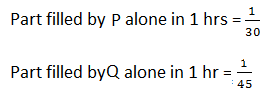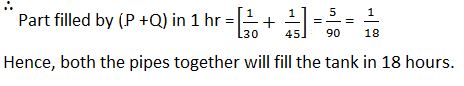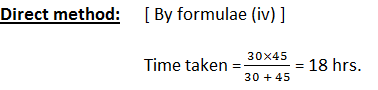Example 2 : A pipe can fill a tank in 25 hrs. Due to a leakage in the bottom, it is filled in 50 hrs. If the tank is full, how much time will the leak take to empty it?

Solution :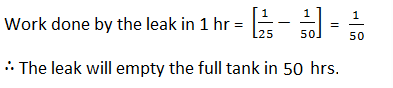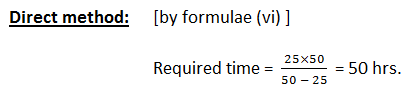Example: Two pipes A and B can fill a tank in 16 minutes and 24 minutes respectively. If both the pipes are opened simultaneously, after how much time should B be closed so that that the tank is full in 12 minutes?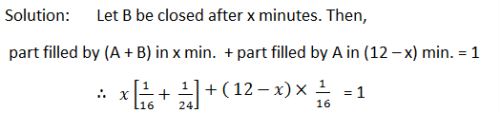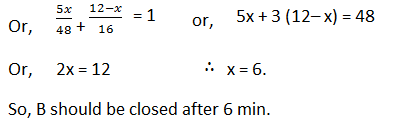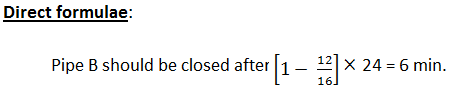Offer running on EduRev: Apply code STAYHOME200 to get INR 200 off on our premium plan EduRev Infinity!

## Quantitative Aptitude for Competitive Examinations

34 videos|34 docs|143 tests

,

,

,

,

,

,

,

,

,

,

,

,

,

,

,

,

,

,

,

,

,

;